# The Normal Distribution l The Standard Normal Distribution

• Slides: 10The Normal Distribution l. The Standard Normal Distribution l. The General Normal Distribution l. Sampling DistributionsThe Standard Normal Distribution Mean 0, Standard Deviation 1 l 68% of the data between -1 and 1. l 95% of the data between -2 and 2. l 99. 7% of the data between -3 and 3. l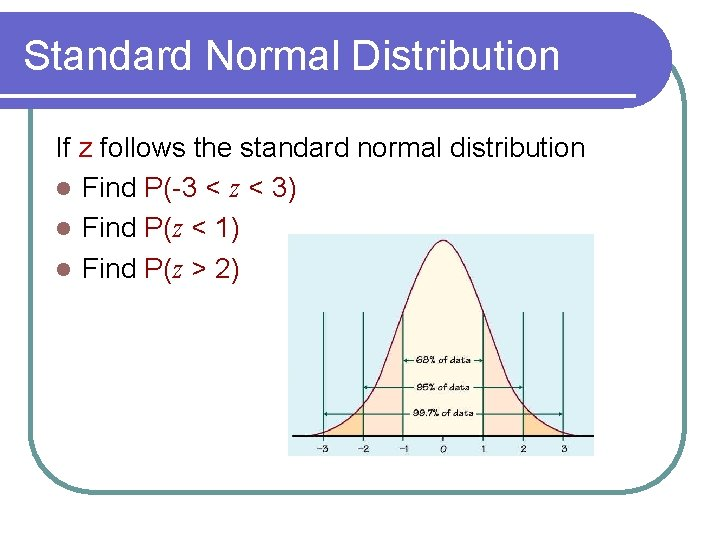Standard Normal Distribution If z follows the standard normal distribution l Find P(-3 < z < 3) l Find P(z < 1) l Find P(z > 2)Using the TI 83/84 For any value of z, we can find the probability with the TI 83/84: 2 nd VARS (DISTR) then normalcdf(a, b) where a is the lower bound and b is the upper bound. To find P(z < b) type in normalcdf(-99999, b). To find P(z > a) type in normalcdf(a, 99999). A. P(0. 21 < z < 1. 18) B. P(z >0. 57) C. P(z < 1. 34) D. P(z >-1. 24)Using the TI 83/84 For any value probability p, we can find the corresponding z such that the area to the left of z is p with the. TI 83/84: 2 nd VARS(DISTR) then inv. Norm(p) A. Find a such that P(z < a) = 0. 38. B. Find a such that P(z > a) = 0. 17.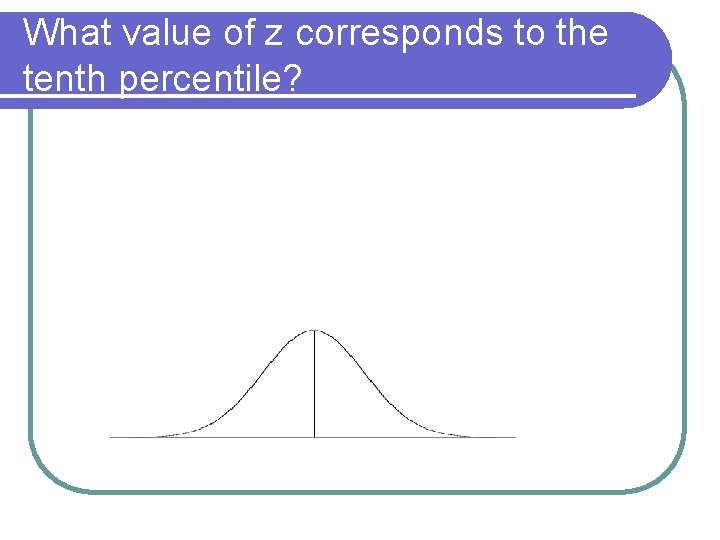What value of z corresponds to the tenth percentile?Review of the z-score Define the z-score as: The z-score tells us how many standard deviations away from the mean the value of x is. It allows us to convert from a general normal distribution to the standard normal distribution.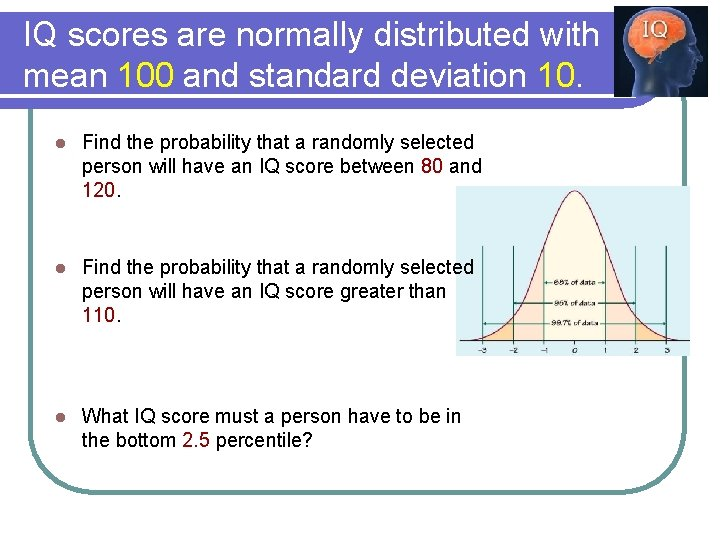IQ scores are normally distributed with mean 100 and standard deviation 10. l Find the probability that a randomly selected person will have an IQ score between 80 and 120. l Find the probability that a randomly selected person will have an IQ score greater than 110. l What IQ score must a person have to be in the bottom 2. 5 percentile?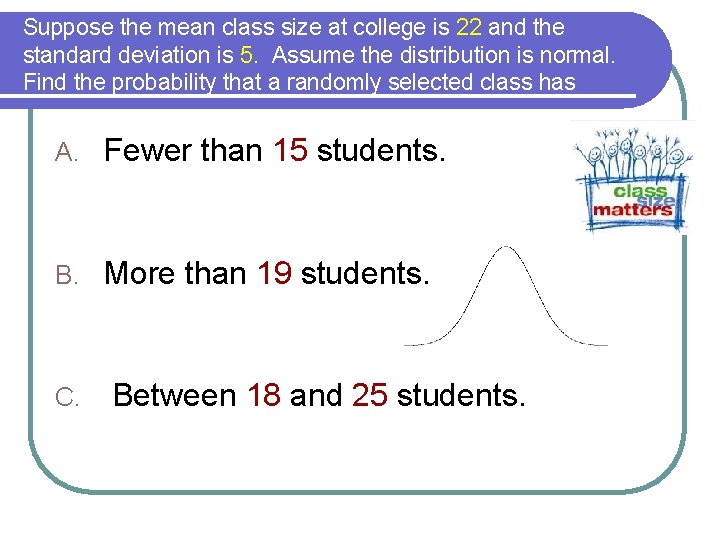Suppose the mean class size at college is 22 and the standard deviation is 5. Assume the distribution is normal. Find the probability that a randomly selected class has A. Fewer than 15 students. B. More than 19 students. C. Between 18 and 25 students.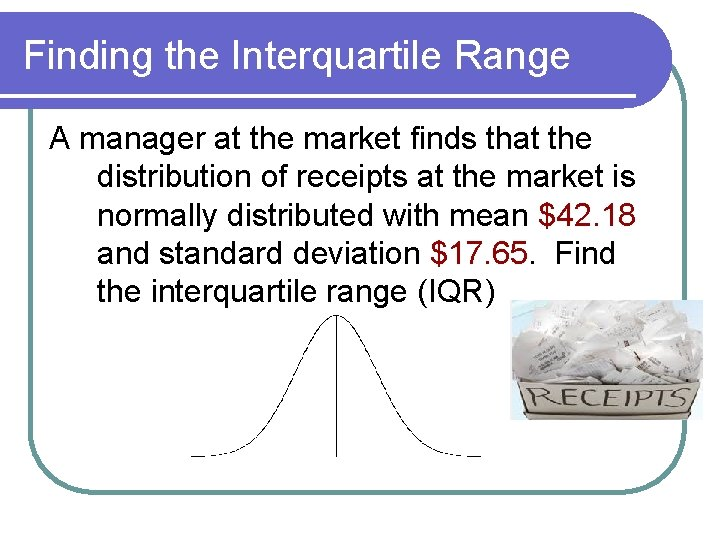Finding the Interquartile Range A manager at the market finds that the distribution of receipts at the market is normally distributed with mean \$42. 18 and standard deviation \$17. 65. Find the interquartile range (IQR)